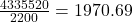## (b) How many days of a diet of 2200 large calories are equivalent to the gravitational energy change from sea level to the top of Mount Ever

Question

(b) How many days of a diet of 2200 large calories are equivalent to the gravitational energy change from sea level to the top of Mount Everest, 8848 m above sea level? Assume your mass is 50 kg. (The body is not anywhere near 100% efficient in converting chemical energy into change in altitude. Also note that this is in addition to your basal metabolism.)

in progress 0
4 weeks 2021-08-19T12:57:46+00:00 1 Answers 0 views 0

1971 days.

Explanation:

As given in the question,

The calories taken per day = 2200

We first need to convert it into Joule, i.e. the SI unit of energy.

Since 1 cal = 4.2 Joule So, 2200 cal = 2200 × 4.2 = 9240 Joules.

Now we have to calculate the gravitational energy change.

As we know the gravitation energy or gravitational potential energy at any point is calculated by multiplying mass(in kg) with the height at that point and acceleration due to gravity.

The gravitational energy at the sea level = 50 × 0 × 9.8 = 0 Joule

Gravitational potential energy at height 8848 m = 50 × 8848 × 9.8 = 4335520 Joules.

Gravitational energy change = 4335520 – 0 = 4335520 Joules.

Number of days required to get such amount of energy =Rounding off to the nearest whole number we get required number of days = 1971 days.